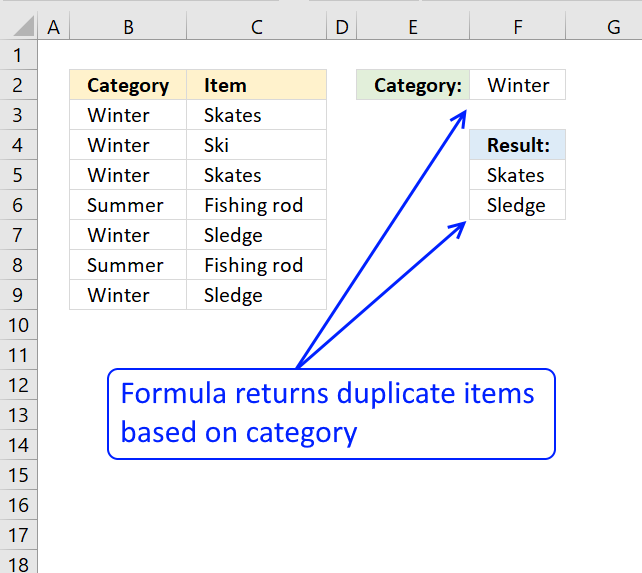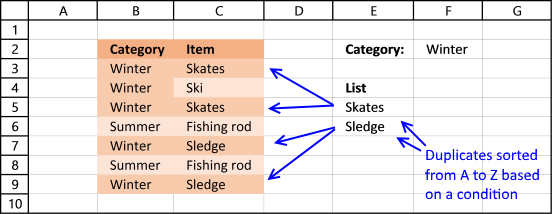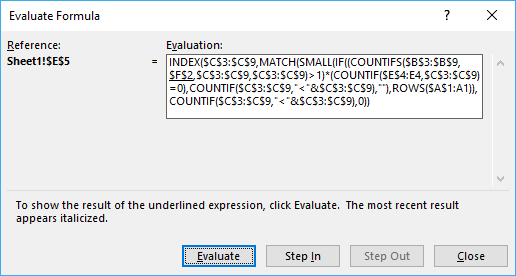Author: Oscar Cronquist Article last updated on February 26, 2018The following image shows you a data set in column B and C. The formula in cell E2 extracts a list of duplicates sorted from A to Z based on a condition in cell F2."Fishing rod" is a duplicate value in column C but it is not in category "Winter", however "Skates" and "Sledge" are duplicates and in category "Winter.

Array formula in cell E5:

=INDEX(\$C\$3:\$C\$9, MATCH(SMALL(IF((COUNTIFS(\$B\$3:\$B\$9, \$F\$2, \$C\$3:\$C\$9, \$C\$3:\$C\$9)>1)*(COUNTIF(\$E\$4:E4, \$C\$3:\$C\$9)=0), COUNTIF(\$C\$3:\$C\$9, "<"&\$C\$3:\$C\$9), ""), ROWS(\$A\$1:A1)), COUNTIF(\$C\$3:\$C\$9, "<"&\$C\$3:\$C\$9), 0))

The following article shows you how to filter unique distinct values sorted alphabetically and based on a condition:

Unique distinct list sorted alphabetically based on a condition

The array formula in cell E6 filters values in column C based on value in cell E3, the output is […]

### How to enter array formula

1. Copy (Ctrl + c) above formula
2. Double click on cell E5
3. Paste formula to cell E5
4. Press and hold CTRL + SHIFT simultaneously
5. Press Enter once
6. Release all keys

The formula now looks like this: {=arrayformula}

Don't enter the curly brackets yourself, they appear automatically.

How to enter an array formula

Array formulas allows you to do advanced calculations not possible with regular formulas.

### Explaining formula in cell E5

You can easily follow along, select cell E5. Go to tab "Formulas" on the ribbon and click "Evaluate Formula" button.Then click "Evaluate" button to move to next step.

#### Step 1 - Check if category is right and return count

COUNTIFS(\$B\$3:\$B\$9, \$F\$2, \$C\$3:\$C\$9, \$C\$3:\$C\$9)>1

becomes

COUNTIFS(\$B\$3:\$B\$9, \$F\$2, \$C\$3:\$C\$9, \$C\$3:\$C\$9)>1

becomes

COUNTIFS({"Winter"; "Winter"; "Winter"; "Summer"; "Winter"; "Summer"; "Winter"},"Winter",{"Skates"; "Ski"; "Skates"; "Fishing rod"; "Sledge"; "Fishing rod"; "Sledge"},{"Skates"; "Ski"; "Skates"; "Fishing rod"; "Sledge"; "Fishing rod"; "Sledge"})>1

and returns

{2;1;2;0;2;0;2}

How to use the COUNTIFS function

Checks multiple conditions against the same number of cell ranges and counts how many times all criteria are met.

#### Step 2 - Check if count is larger than 1

This allows us to filter duplicate values.

COUNTIFS(\$B\$3:\$B\$9, \$F\$2, \$C\$3:\$C\$9, \$C\$3:\$C\$9)>1

becomes

{2;1;2;0;2;0;2}>1

and returns {TRUE; FALSE; TRUE; FALSE; TRUE; FALSE; TRUE}

#### Step 3 - Count previous values in list

COUNTIF(\$E\$4:E4, \$C\$3:\$C\$9)

returns {0;0;0;0;0;0;0}

How to use the COUNTIF function

Counts the number of cells that meet a specific condition.

#### Step 4 - Check if they have not been shown before

This makes sure that previous values in column E are not repeated.

COUNTIF(\$E\$4:E4, \$C\$3:\$C\$9)>0

becomes

{0;0;0;0;0;0;0}=0

and returns {TRUE; TRUE; TRUE; TRUE; TRUE; TRUE; TRUE}

#### Step 5 - Multiply arrays

(COUNTIFS(\$B\$3:\$B\$9, \$F\$2, \$C\$3:\$C\$9, \$C\$3:\$C\$9)>1)*(COUNTIF(\$E\$4:E4, \$C\$3:\$C\$9)=0)

becomes

{TRUE; FALSE; TRUE; FALSE; TRUE; FALSE; TRUE}*{TRUE; TRUE; TRUE; TRUE; TRUE; TRUE; TRUE}

and returns {1;0;1;0;1;0;1}

#### Step 6 - Build an array of sort ranking numbers

COUNTIF(\$C\$3:\$C\$9, "<"&\$C\$3:\$C\$9) returns {2;4;2;0;5;0;5}

#### Step 7 - Return corresponding sort rank number

IF((COUNTIFS(\$B\$3:\$B\$9, \$F\$2, \$C\$3:\$C\$9, \$C\$3:\$C\$9)>1)*(COUNTIF(\$E\$4:E4, \$C\$3:\$C\$9)=0), COUNTIF(\$C\$3:\$C\$9, "<"&\$C\$3:\$C\$9), "")

becomes

IF({1;0;1;0;1;0;1},{2;4;2;0;5;0;5}, "")

and returns {2;"";2;"";5;"";5}

How to use the IF function

Checks if a logical expression is met. Returns a specific value if TRUE and another specific value if FALSE.

#### Step 8 - Find the n-th smallest number

SMALL(IF((COUNTIFS(\$B\$3:\$B\$9, \$F\$2, \$C\$3:\$C\$9, \$C\$3:\$C\$9)>1)*(COUNTIF(\$E\$4:E4, \$C\$3:\$C\$9)=0), COUNTIF(\$C\$3:\$C\$9, "<"&\$C\$3:\$C\$9), ""), ROWS(\$A\$1:A1))

becomes

SMALL({2;"";2;"";5;"";5}, ROWS(\$A\$1:A1))

becomes

SMALL({2;"";2;"";5;"";5}, 1)

and returns 2.

How to use the SMALL function

The SMALL function lets you extract a number in a cell range based on how small it is compared to the other numbers in the group.

#### Step 9 - Get position of value in array

MATCH(SMALL(IF((COUNTIFS(\$B\$3:\$B\$9, \$F\$2, \$C\$3:\$C\$9, \$C\$3:\$C\$9)>1)*(COUNTIF(\$E\$4:E4, \$C\$3:\$C\$9)=0), COUNTIF(\$C\$3:\$C\$9, "<"&\$C\$3:\$C\$9), ""), ROWS(\$A\$1:A1)), COUNTIF(\$C\$3:\$C\$9, "<"&\$C\$3:\$C\$9), 0)

becomes

MATCH(2, COUNTIF(\$C\$3:\$C\$9, "<"&\$C\$3:\$C\$9), 0)

becomes

MATCH(2, {2;4;2;0;5;0;5}, 0)

and returns 1.

How to use the MATCH function

Identify the position of a value in an array.

#### Step 10 - Return value in data set based on coordinate

INDEX(\$C\$3:\$C\$9, MATCH(SMALL(IF((COUNTIFS(\$B\$3:\$B\$9, \$F\$2, \$C\$3:\$C\$9, \$C\$3:\$C\$9)>1)*(COUNTIF(\$E\$4:E4, \$C\$3:\$C\$9)=0), COUNTIF(\$C\$3:\$C\$9, "<"&\$C\$3:\$C\$9), ""), ROWS(\$A\$1:A1)), COUNTIF(\$C\$3:\$C\$9, "<"&\$C\$3:\$C\$9), 0))

becomes

INDEX(\$C\$3:\$C\$9, 1)

becomes

INDEX({"Skates";"Ski";"Skates";"Fishing rod";"Sledge";"Fishing rod";"Sledge"}, 5)

and returns "Skates" in cell E5.

How to use the INDEX function

Gets a value in a specific cell range based on a row and column number.